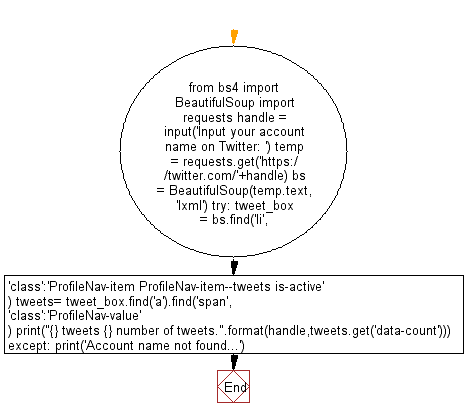# Python Web Scraping: Count number of tweets by a given Twitter account

## Python Web Scraping: Exercise-19 with Solution

Write a Python program to count number of tweets by a given Twitter account.

Sample Solution:-

Python Code:

``````from bs4 import BeautifulSoup
import requests

bs = BeautifulSoup(temp.text,'lxml')

try:
tweet_box = bs.find('li',{'class':'ProfileNav-item ProfileNav-item--tweets is-active'})
tweets= tweet_box.find('a').find('span',{'class':'ProfileNav-value'})
print("{} tweets {} number of tweets.".format(handle,tweets.get('data-count')))

except:
```
```

Output

```Input your account name on Twitter:  @prasanta
@prasanta tweets 36 number of tweets.
```

Flowchart:Python Code Editor:

Have another way to solve this solution? Contribute your code (and comments) through Disqus.

What is the difficulty level of this exercise?

﻿

## Python: Tips of the Day

Checks if the given number falls within the given range.

Example:

```def tips_range(n, start, end = 0):
return start <= n <= end if end >= start else end <= n <= start
print(tips_range(2, 4, 6))
print(tips_range(4, 8))
print(tips_range(1, 3, 5))
print(tips_range(1, 3))
```

Output:

```False
True
False
True
```